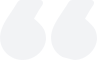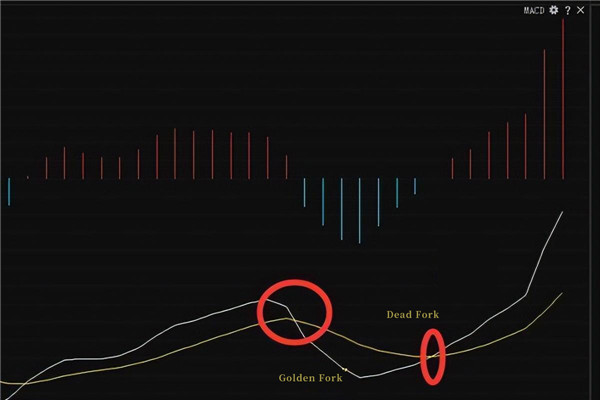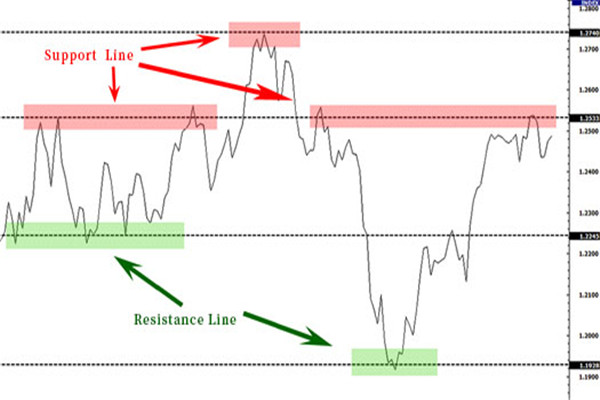# Detailed Explanation of MACD Calculation Formula

2023-06-01Summary:

When the MACD line crosses the signal line from bottom to top, it is usually considered a buy signal, while when the MACD line crosses the signal line from top to bottom, it is considered a sell signal.

MACD should first calculate the fast (usually 12 days) moving average value and the slow (usually 26 days) moving average value in the application. Use these two values as the basis for measuring the "difference value" between the two (fast and slow lines).

The so-called "difference value" (DIF) refers to the 12 day EMA value minus the 26 day EMA value. Therefore, in the sustained upward trend, the 12th EMA is above the 26th EMA. The positive deviation value (+DIF) between them will become larger and larger. On the contrary, in a downward trend, the difference value may become negative (-DIF) and also increase.

As for the degree to which the positive or negative deviation value needs to be reduced when the market starts to turn around, it is truly a signal of market reversal. The reversal signal of MACD is defined as the 9-day moving average (9-day EMA) of the "difference value".The calculation formula for MACD indicators involves three parts: a fast line, a slow line, and a bar chart.

1. Calculate the fast line (EMA12).

Firstly, it is necessary to calculate the 12-day average index (EMA12) of the closing price, which is:

EMA12=Previous day EMA12 x 11/13+ Today's closing price x 2/13

Among them, 11/13 and 2/13 are weighting coefficients that can be adjusted according to different analysis requirements.

2. Calculate the slow line (EMA26).

Next, it is necessary to calculate the 26-day average index (EMA26) of the closing price, which is:

EMA26=Previous day EMA26 x 25/27+ Today's closing price x 2/27

Similarly, 25/27 and 2/27 are weighted coefficients.

3. Calculate bar charts (DIF and DEA).

Finally, the difference between the fast and slow lines can be used as a bar chart (DIF) to measure price momentum and trend changes while also calculating the 9-day moving average (DEA) of the DIF, which is:

DEA=previous day's DEA x 8/10 + today's DIF x 2/10

Finally, the calculation formula for MACD indicators can be expressed as:

MACD=DIF-DDEA

Its IF is the difference between the fast line and the slow line, DEA is the 9-day moving average of DIF, and MACD is the bar chart. It should be noted that the value of the MACD index can be positive, negative, or zero, and its size and direction reflect the change and trend intensity of market prices.### MACD Golden Fork Dead Fork Strategy Introduction

This article introduces the MACD strategy, pointing out its simple principles and trend judgment methods.

2023-09-22### Support and Resistance Line Analysis Key Takeaways

The resistance line marks a stubborn stock price barrier, and the support line signals a persistent price floor, both resisting multiple breakouts.

2023-09-21### Mastering RSI Bottom Deviation for Effective Use

In the stock market, it is crucial to understand and apply the RSI (relative strength index) bottom deviation.

2023-09-20